# Y4 Maths Task- Wednesday 25th March

Fractions and decimals

You might need to work out on paper and then write it in your reply. If you have a printer, you could print it out and write your answers on there first.

This activity should take around an hour.

Click on the blue link to see the work more clearly.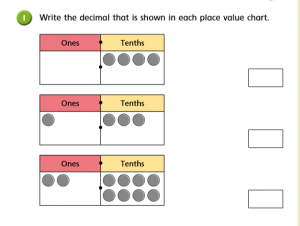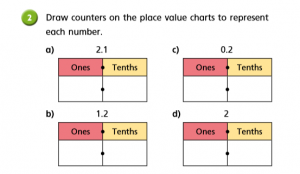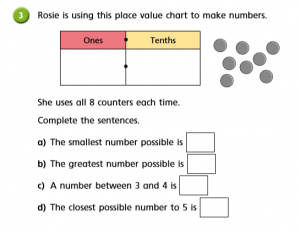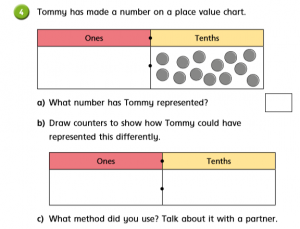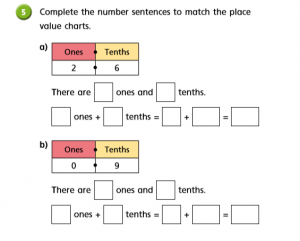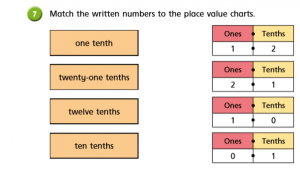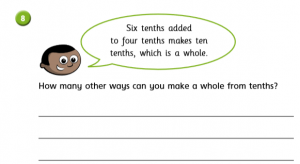maths lesson 3

### 14 thoughts on “Y4 Maths Task- Wednesday 25th March”

•25th March 2020 at 11:39 am

1: 1: 0.4 2:1.3 3: 2.8
2: a: 2 ones and 1 tenth. b: 1 one and 2 tenths. c: 0 ones and 2 tenths. d: 2 ones and 0 tenths.
3: a: 0.8 b: 8.0 c: 3.5 d 5.3.
4: a: 1.3 b: 1 one and 3 tenths. c: There was no specific amount of counters so I knew that all I had to do was just put one whole and three tenths and if you put them together, you get 1.3. (The number tommy made)
5: a: There are 2 ones and 6 tenths. 2 ones plus 6 tenths equals 2 plus 0.6 Equals 2.6. b: There are 0 ones and 9 tenths. 0 ones plus 9 tenths equals 0 plus 0.9 equals 0.9.
6: a: 3 ones and 2 tenths. b: 5 ones and 2 tenths.
7: one tenth-0 ones and 1 tenth. Twenty one tenths-2 ones and 1 tenth. Twelve tenths-1 one and 2 tenths. Ten tenths-1 one and 0 tenths.
8: 1 plus 9. 2 plus 8. 3 plus 7. 4 plus 6. 5 plus 5. 6 plus 4. 7 plus 3. 8 plus 2. 9 plus 1.

•25th March 2020 at 12:07 pm

Great job Ben. Hope you are enjoying the sunshine

•25th March 2020 at 12:06 pm

1.
0.4
1.3
2.8

2.
a)2 ones and 1 tenths
b)1 ones and 2 tenths
c)0 ones and 2 tenths
d)2 ones and 0 tenths

3.
a)the smallest number possible is 8
b)the greatest number possible is 80
c)a number between 3 and 4 is 4
d)the closest possible number to 5 is 4

4.
split them but then there would be 1 left so it would be fair

5.
a)there are 2 ones and 6 tenths.
2ones+6tenths=2+60=62
b)there are 0 ones and 9 tenths.
0 ones+9tenths=0+90=90

6.
a)3.2
b)5.2
c)0.2

7.
one tenths

one tenth = 0 1
twenty-one tenths = 1 2
twelve tenths = 2 1
ten tenths = 0 10

8.
you can do 5 tenths +5 tenths=whole

•25th March 2020 at 3:07 pm

1) 0.4 1.3 2.8

2) 2.1 1.2 0.2 2.0

3) The smallest number possible is 1.7
The greatest number possible is 8.0
A number between 3 and 4 is 2.6
The closest possible number to 5 is 5.3

4)Tommy has represented the number 1.3

b) 0.000

•25th March 2020 at 3:23 pm

Well done Maisie. I hope you having a good day

•27th March 2020 at 12:22 pm

1. 0.4 1.3 2.8
2. 2.1 1.2 0.2 2.0
3.0.8 8.0 3.5 5.3
4. a) 1.3 b)1 one and three tenth’s c)I worked it out by counting the number then working it out.
5.a 2 ones and 6 tenth’s = 2 ones + 0.6 tenths =2.6 b) 0 ones and 9 tenths or 0+ 0.9=0.9
6.a 3.2 b 5.2 c 0.2
7. 1 tenth = 0.1 21 tenths =2.1 twelve tenths=1.2 10 tenths=1.0
8. there’s 5 ways.

•27th March 2020 at 12:49 pm

You have a great understanding of decimals. Excellent Owen

•28th March 2020 at 7:04 pm

1.
0.4
1.3
2.8

2.
a) 2 ones and 1 tenths
b) 1 ones and 2 tenths
c) 0 ones and 2 tenths
d) 2 ones and 0 tenths

3.
a) The smallest number possible is 0.8
b) The greatest number possible is 8.0
c) A number between 3 and 4 is 3.5
d) The closest possible number to 5 is 5.3

4.
a) 1.3
b) 1 ones 3 tenths

5.
a) There are 2 ones and 6 tenths.
2 ones+ 6 tenths = 2+ 0.6 = 2.6
b) There are 0 ones and 9 tenths.
0 ones + 9 tenths = 0 + 0.9 = 0.9

6.
a) 3.2
b) 5.2
c) 0.2

7.
one tenths

one tenth = 0 1
twenty-one tenths = 2 1
twelve tenths = 1 2
ten tenths = 1 0

8.
0.6 + 0.4 = 1
0.1 + 0.9 = 1
0.2 + 0.8 = 1
0.3 + 0.7 = 1
0.4 + 0.6 = 1
0.5 + 0.5 = 1

•29th March 2020 at 3:11 pm

Well done. You have completed some tricky problem solving tasks here :).

•30th March 2020 at 4:21 pm

1a)0.4
b)1.3
c)2.8
2a)2.1
b)1.2
c)0.2
d)2.0
3a) 0.8
b)8.0
c)3.5
d)4.4
4) 1.3 , 1 counter in ones and 3 counters in tenths
5) There are 2 ones and 6 tenths, 2 ones +6 tenths=20+6=26 tenths
There are 0 ones and 9 tenths, 0 ones +9 tenths= 0+9= 9 tenths
6) 3.2, 5.2
7) one tenth= 0.1
twenty-one tenths=2.1
twelve tenths= 1.2
ten tenths=1.0
8)5/10+5/10= 1
e.g. 9/10+1/10=1

•30th March 2020 at 7:11 pm

Thank you Damian great understanding.

•23rd April 2020 at 3:53 pm

1
0.4
1.3
2.8

2.
a) ** ones and * tenths
b) * ones and ** tenths
c) 0 ones and ** tenths
d) * ones and 0 tenths

3.
a) 0.8
b) 8
c) 3.5
d) 5.3

4.
a) 1.3
b) * ones *** tenths

5.
a) There are 2 ones and 6 tenths
2 ones + 6 tenths = 2+ 0.6 = 2.6
b) There are 0 ones and 9 tenths.
0 ones + 9 tenths = 0 + 0.9 = 0.9

6.
a) 3.2
b) 5.2
c) 0.2

7.
one tenth = 0 & 1
twenty-one tenths = 2 & 1
twelve tenths = 1 & 2
ten tenths = 1 & 0
8.
0.1 + 0.9 = 1,
0.2 + 0.8 = 1,
0.3 + 0.7 = 1,
0.4 + 0.6 = 1,
0.5 + 0.5 = 1 ,
0.6 + 0.4 = 1,
0.7 + 0.3 = 1,
0.8 + 0.2 = 1,
0.9 + 0.1 = 1

•12th May 2020 at 8:17 pm
•